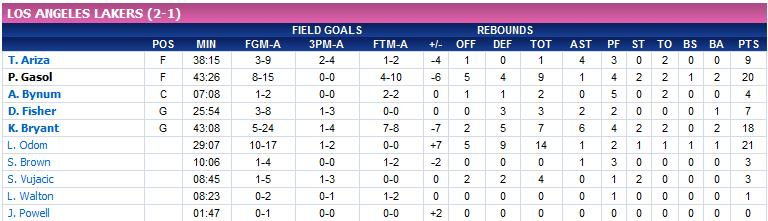# The mode

The mode of a set of information is the value that appears with a major frequency.

A dice is thrown ten times and the results are the following:

$$1, 3, 2, 5, 4, 2, 6, 2, 3, 6$$\$

The table of absolute frequencies is:

 Result of the dice Absolute frequency $$1$$ $$1$$ $$2$$ $$3$$ $$3$$ $$2$$ $$4$$ $$1$$ $$5$$ $$1$$ $$6$$ $$2$$

It is easy to see that the mode is $$2$$, for it is the most frequent result.

The statistics of the players of Los Angeles Lakers in the first match of the finals of the NBA are the following:a) What is the mode of scored points (PTS) among the Lakers players?

We can see in the last column, the points column, the only value that recurs is 3 points (those scored by S. Brown and S. Vujacic). And so, the mode is $$3$$.

b) And the mode of personal fouls (PF)?

The relative table of the number of personal fouls is:

 Personal fouls Absolute frequency $$0$$ $$1$$ $$1$$ $$2$$ $$2$$ $$2$$ $$3$$ $$2$$ $$4$$ $$2$$ $$5$$ $$1$$

We can see that the values $$1, 2, 3$$ and $$4$$ recur twice, that is, two players made $$1$$ foul, $$2$$ made $$2$$ fouls, etc. And so, the mode of the personal fouls is $$1, 2, 3$$ and $$4$$.